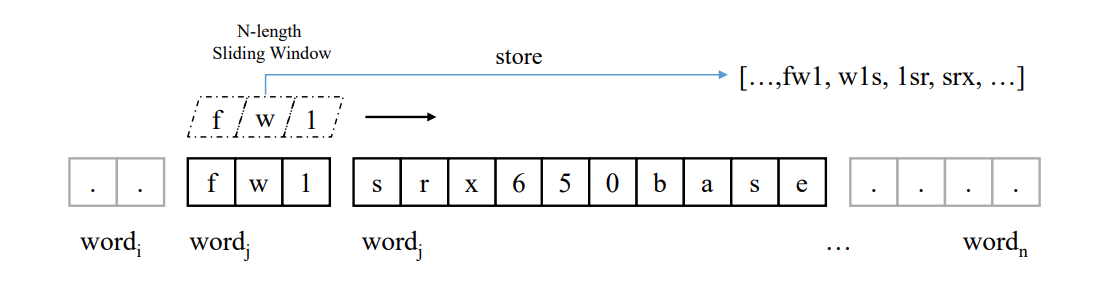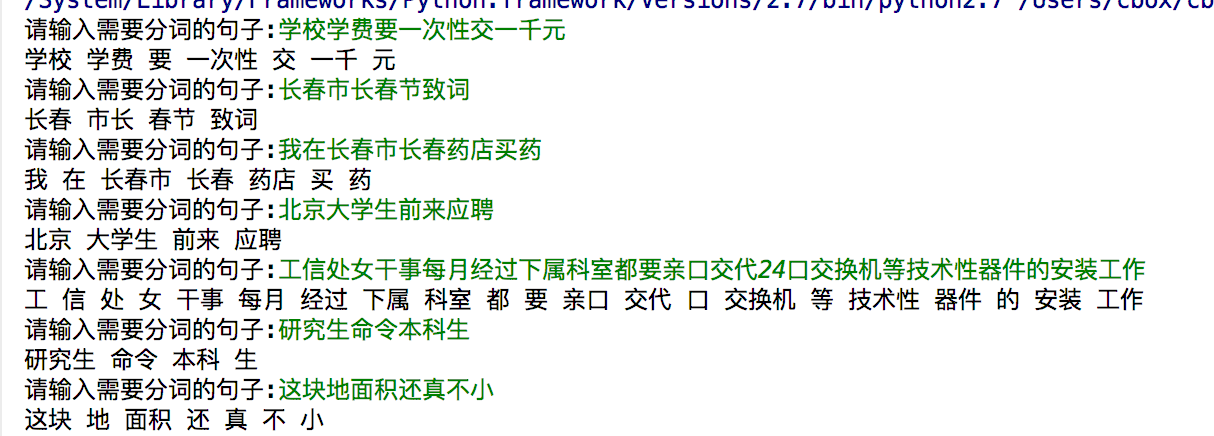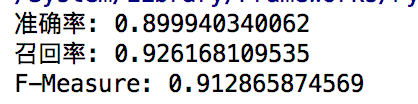• 本篇博客使用Python编程语言实现基于概率最大化的中文分词算法。 2 理论描述 基于概率的自动分词算法 (1)基本思想：选择概率最大的分词路径作为最优结果 (2)利用字与字间、词与词间的同现频率作为分词的依据， ...
1 概述
汉语自动分词是把没有明显分界标志的字串切分为词串。包括：标点符号、数字、数学符号、各种 标记、人名、地名、机构名等未登录词的识别。本篇博客使用Python编程语言实现基于概率最大化的中文分词算法。
2 理论描述
基于概率的自动分词算法
(1)基本思想：选择概率最大的分词路径作为最优结果
(2)利用字与字间、词与词间的同现频率作为分词的依据， 可以没有建立好的词典。需要大规模的训练文本, 用来训练模型参数。
(3)优点：不受应用领域的限制；
(4) 缺点：训练文本的选择将影响分词结果。
3 算法描述
(1)对一个待分词的字串S,按照从左到右的顺序取出全部候选词$W_1$,$W_2$,…,$W_i$,$W_n$;
(2)计算每个候选词的概率值P($W_i$ ),记录每个候选词的全部左邻词;
(3) 计算每个候选词的累计概率，累计概率最大的候选词为最佳左邻词;
(4)如果当前词Wn是字串的尾词,且累计概率P’($W_n$)最大，则 是S的终点词；
(5)从$W_n$开始，按照从右到左顺序，依次将每个词的最佳左邻词输出，即S的分词结。
4 详例描述
输入：结合成分子时
第一步：遍历整句话，找出候选词和在语料库中的词频

候选词
词频

结
0.0037%

结合
0.0353%

合
0.0049%

合成
0.0006%

成
0.0423%

成分
0.0023%

分
0.0312%

分子
0.0038%

子
0.0010%

时
0.1043%

第二步：找出备选左邻词

候选词
备选左邻词

结

结合

合
结

合成
结

成
结合、合

成分
结合、合

分
合成、成

分子
合成、成

子
成分、分

时
分子、子

第三步：计算累计概率，选出最佳左邻词

候选词
累计概率
备选左邻词
最佳左邻词

结
0.0037%

结合
0.0353%

合
0.00001813%
结
结

合成
0.00000222%
结
结

成
0.00149319%
结合、合
结合

成分
0.00008119%
结合、合
结合

分
0.0000465875%
合成、成
成

分子
0.00000567412%
合成、成
成

子
0.00000008119%
成分、分
成分

时
0.000000591811%
分子、子
分子

第四步：输出结果

结合/成/分子/时

5 实现代码
def load_dict():
"""
加载字典
:return:返回字典==>"词:频率"
"""
dic_file = open("WordFrequency.txt", 'r', encoding='utf-8')
freq_dict = {}
for l in dic_file:
freq_dict[l.split(',')] = l.split(',').strip()
dic_file.close()
return freq_dict

def find_word_in_dict(s):
"""
在字典中查找候选词
:param s: 输入句子
:return: 返回字典==>"词:词频|候选左邻词1/候选左邻词2"
"""
result = {}
for index in range(0, len(s)):  # 遍历所有字
for wordLen in range(0, len(s) - index):  # 遍历该字的所有可能词
seg_word = s[index:index + wordLen + 1]
if seg_word in freq_dict.keys():
# 找到候选词，找其左邻词
left_words = ''
for word_len in range(index, 0, -1):  # 在之前的候选词列表里找左邻词（最大词长开始）
for k in result.keys():
if s[index - word_len:index] == k.split('-'):
left_words += str(index - word_len) + '-' + s[index - word_len:index] + '/'
# 返回候选词及其语料库词频和候选左邻词
result[str(index) + '-' + seg_word] = freq_dict[seg_word] + '|' + left_words
return result

def cl_probability(words_dict):
"""
计算累加概率并选择最佳左邻词
:param words_dict: "词:词频|候选左邻词1/候选左邻词2"
:return:返回新字典==>"词:累计概率|最佳左邻词"
"""
for k, v in words_dict.items():
freq = v.split('|')[:-1]
left_words = v.split('|')
if left_words == '':
continue
else:
left_word = left_words.split("/")
max_left_p = 0.0
which_word = ''
for num in range(0, len(left_word) - 1):
curr_left_word_p = float(words_dict[left_word[num]].split('|')[:-1])
if curr_left_word_p > max_left_p:  # 比较当前左邻词的累计概率
max_left_p = curr_left_word_p
which_word = left_word[num]
curr_max_p = float(max_left_p) * float(freq)
# 用最大累计概率替换原来的词频,用最佳左邻词替换候选左邻词
words_dict[k] = v.replace(freq, str(curr_max_p)).replace(left_words, which_word)
return words_dict

def seg(sentence):
"""
接收输入，调用函数并输出
:param sentence:
:return: 分词后的句子
"""
words_dict = find_word_in_dict(sentence)
best_words_dict = cl_probability(words_dict)
seg_line = ''
keys = list(best_words_dict.keys())
key = keys[-1]
while key != '':
seg_line = key.split('-') + '/ ' + seg_line
key = best_words_dict[key].split('|')
return seg_line

if __name__ == '__main__':
print("概率最大化分词-请输入待切分句子：")
input_str = input()
print(seg(input_str))


6 总结
这里使用的词典格式是词，语料库中出现的次数，词频，大家可以根据实际字典修改loadDict函数。

和,10919,0.9737%
是,9847,0.8781%
“,7970,0.7107%
”,7943,0.7083%
一,7335,0.6541%

找左邻词要考虑子串重复的情况，这里想了很久，如不处理，在输入为分子结合成分子时，分子分子分子时会有Bug。因为字典的键值重复了，所以后面出现的分子的值会把前面分子的值覆盖掉，因此，我在写入键和左邻词的时候加上了首字的index使其具有唯一性。不过这样在后面的切片时要更为细心，多多调试，如果是Java的话估计我已经放弃了hh…
处理前：

{‘分’: ‘0.0312%|合成/成/’, ‘分子’: ‘0.0038%|合成/成/’, ‘子’: ‘0.0010%|成分/分/’, ‘结’: ‘0.0037%|分子/子/’, ‘结合’: ‘0.0353%|分子/子/’, ‘合’: ‘0.0049%|结/’, ‘合成’: ‘0.0006%|结/’, ‘成’: ‘0.0423%|结合/合/’, ‘成分’: ‘0.0023%|结合/合/’, ‘时’: ‘0.1043%|分子/子/’}

处理后：

{‘0-结’: ‘0.0037%|’, ‘0-结合’: ‘0.0353%|’, ‘1-合’: ‘0.0049%|0-结/’, ‘1-合成’: ‘0.0006%|0-结/’, ‘2-成’: ‘0.0423%|0-结合/1-合/’, ‘2-成分’: ‘0.0023%|0-结合/1-合/’, ‘3-分’: ‘0.0312%|1-合成/2-成/’, ‘3-分子’: ‘0.0038%|1-合成/2-成/’, ‘4-子’: ‘0.0010%|2-成分/3-分/’, ‘5-时’: ‘0.1043%|3-分子/4-子/’}

算法效率影响因素主要考虑如何寻找候选左邻词，考虑过从后往前遍历保留候选词，不过在寻找左邻词时开销比较大，索性在正序找候选词时一并将候选左邻词一并保存，之后通过更新字典值的方法计算累计概率。最后在计算的时候注意一下%的处理，str转float等一些语法的问题就可以啦。
最后一点要注意的是，在某些情况下，随着累乘次数的增加，累计概率的值可能会小于python的float的精度，这里给出的解决思路是用lg将累乘问题变成累加问题，只需要修改clProbabilty函数即可。


展开全文• 本次实验内容是基于词典的双向匹配算法的中文分词算法的实现。使用正向和反向最大匹配算法对给定句子进行分词，对得到的结果进行比较，从而决定正确的分词方法。 算法描述 正向最大匹配算法 先设定扫描的窗口大小...
摘要
本次实验内容是基于词典的双向匹配算法的中文分词算法的实现。使用正向和反向最大匹配算法对给定句子进行分词，对得到的结果进行比较，从而决定正确的分词方法。
算法描述
正向最大匹配算法
先设定扫描的窗口大小maxLen（最好是字典最长的单词长度），从左向右取待切分汉语句的maxLen个字符作为匹配字段。查找词典并进行匹配。若匹配成功，则将这个匹配字段作为一个词切分出来，并将窗口向右移动这个单词的长度。若匹配不成功，则将这个匹配字段的最后一个字去掉，剩下的字符串作为新的匹配字段，进行再次匹配，重复以上过程，直到切分出所有词为止。
反向最大匹配算法
该算法是正向的逆向算法，区别是窗口是从后向左扫描，若匹配不成功，则去掉第一个字符，重复上述的匹配步骤。
双向最大匹配算法
双向最大匹配法是将正向最大匹配法得到的分词结果和逆向最大匹配法的到的结果进行比较，从而决定正确的分词方法。定义的匹配规则如下：

如果正反向匹配算法得到的结果相同，我们则认为分词正确，返回任意一个结果即可。
如果正反向匹配算法得到的结果不同，则考虑单字词、非字典词、总词数数量的数量，三者的数量越少，认为分词的效果越好。我们设定一个惩罚分数（score_fmm / score_bmm = 0），例如：正向匹配中单字词数量多于反向匹配，则正向匹配的分值score_fmm += 1。其他两个条件相同。可以根据实际的分词效果调整惩罚分数的大小，但由于没有正确分词的数据，因此惩罚分数都设为1。最后比较惩罚分数，返回较小的匹配结果。

详例描述
以“对外经济技术合作与交流不断扩大。”为例，详细描述算法如下：
窗口大小设为4，句子长度为16，分词列表words = []。

首先是正向匹配。sub_str = ‘对外经济’与词典进行匹配，匹配失败，窗口大小减一。
sub_str = ‘对外经’与词典进行匹配，匹配失败，窗口大小减一。
sub_str = ‘对外’与词典进行匹配，匹配成功，窗口大小恢复为4，向右移动之前匹配词的长度，此时sub_str = ‘经济技术’，将其添加至列表words中。重复上述步骤。
当匹配到最后一个词时，算法停止。
正向匹配结果如下：[‘对外’, ‘经济’, ‘技术’, ‘合作’, ‘与’, ‘交流’, ‘不断’, ‘扩大’, ‘。’]
反向匹配如法炮制，结果如下：[‘对外’, ‘经济’, ‘技术’, ‘合作’, ‘与’, ‘交流’, ‘不断’, ‘扩大’, ‘。’]
正向与反向匹配结果相同，返回任意一个。

代码
加载词典
def read_dict(path):
words_dict = []
with open(path, 'r') as r:
# print(line)
for i in line:
word = i.split(',')
words_dict.append(word)
return words_dict

window_size = 4

正向匹配算法
def fmm(source, words_dict):
len_source = len(source)  # 原句长度
index = 0
words = []  # 分词后句子每个词的列表

while index < len_source:  # 如果下标未超过句子长度
match = False
for i in range(window_size, 0, -1):
sub_str = source[index: index+i]
if sub_str in words_dict:
match = True
words.append(sub_str)
index += i
break
if not match:
words.append(source[index])
index += 1
return words

反向匹配算法
def bmm(source, word_dict):
len_source = len(source)  # 原句长度
index = len_source
words = []  # 分词后句子每个词的列表

while index > 0:
match = False
for i in range(window_size, 0, -1):
sub_str = source[index-i: index]
if sub_str in words_dict:
match = True
words.append(sub_str)
index -= i
break
if not match:
words.append(source[index-1])
index -= 1
words.reverse()  # 得到的列表倒序
return words

双向匹配算法
def bi_mm(source, word_dict):
forward = fmm(source, words_dict)
backward = bmm(source, words_dict)
# 正反向分词结果
print("FMM: ", forward)
print("BMM: ", backward)
# 单字词个数
f_single_word = 0
b_single_word = 0
# 总词数
tot_fmm = len(forward)
tot_bmm = len(backward)
# 非字典词数
oov_fmm = 0
oov_bmm = 0
# 罚分，罚分值越低越好
score_fmm = 0
score_bmm = 0
# 如果正向和反向结果一样，返回任意一个
if forward == backward:
return backward
# print(backward)
else:  # 分词结果不同，返回单字数、非字典词、总词数少的那一个
for each in forward:
if len(each) == 1:
f_single_word += 1
for each in backward:
if len(each) == 1:
b_single_word += 1
for each in forward:
if each not in words_dict:
oov_fmm += 1
for each in backward:
if each not in backward:
oov_bmm += 1
# 可以根据实际情况调整惩罚分值
# 这里都罚分都为1分
# 非字典词越少越好
if oov_fmm > oov_bmm:
score_bmm += 1
elif oov_fmm < oov_bmm:
score_fmm += 1
# 总词数越少越好
if tot_fmm > tot_bmm:
score_bmm += 1
elif tot_fmm < tot_bmm:
score_fmm += 1
# 单字词越少越好
if f_single_word > b_single_word:
score_bmm += 1
elif f_single_word < b_single_word:
score_fmm += 1

# 返回罚分少的那个
if score_fmm < score_bmm:
return forward
else:
return backward

主函数，测试了分词的时间，包括加载词典的时间
if __name__ == '__main__':
start = time.time()
# print(bmm("我正在上自然语言处理课。", words_dict))
# print("result: ", result)
print("BiMM: ", bi_mm("对外经济技术合作与交流不断扩大。", words_dict))
end = time.time()
print("running time: " + str(end - start) + "s")



展开全文• N-Gram 算法是一种单词级别的窗口取词算法，N-Gram（有时也称为N元模型）是自然语言处理中一个非常重要的概念，通常在NLP中，人们基于一定的语料库，可以利用N-Gram来预计或者评估一个句子是否合理。另外一方面，N-...
概述
N-Gram 算法是一种单词级别的窗口取词算法，N-Gram（有时也称为N元模型）是自然语言处理中一个非常重要的概念，通常在NLP中，人们基于一定的语料库，可以利用N-Gram来预计或者评估一个句子是否合理。另外一方面，N-Gram的另外一个作用是用来评估两个字符串之间的差异程度。这是模糊匹配中常用的一种手段。
N-Gram 算法具体过程：

过滤掉文本数据中的标点符号和其他特殊字符；

对所有单词执行小写转换，并删除单词之间的空格、换行符等标志位；

使用长度为 N 的窗口对文本内容执行字符级滑动取词，将结果存入有序列表。

如下图所示程序分为两步：文本过滤、滑动取词
文本过滤
def text_filter(text: str) -> str:
"""
文本过滤器：过滤掉文本数据中的标点符号和其他特殊字符
:param text: 待过滤的文本
:return: 过滤后的文本
"""
result = str()
for t in text:
if t.isalnum():
if t.isalpha():
t = t.lower()
result += str(t)
return result

滑动取词
def slide_word(text: str, l: int = 5) -> list:
"""
滑动取词器
Input: text='abcd',l=2
Output: ['ab','bc','cd']
:param text: 过滤后的文本 （只包含小写数字/字母）
:param l: 滑动窗口长度，默认为 5
:return:
"""
tf = text_filter(text)
result = list()
if len(tf) <= l:
result.append(tf)
return result
for i in range(len(tf)):
word = tf[i:i + l]
if len(word) < l:
break
result.append(word)
return result

测试
if __name__ == '__main__':
banner = 'abcdefghigkLMN*^%\$*   \r\n)021'
print(slide_word(banner))

输出
['abcde', 'bcdef', 'cdefg', 'defgh', 'efghi', 'fghig', 'ghigk', 'higkl', 'igklm', 'gklmn', 'klmn0', 'lmn02', 'mn021']



展开全文字符串 自然语言处理
• mmseg中文分词算法python实现及其优化任务定义实现一个中文分词系统并对其性能做测试。输入输出该分词的训练语料取自人民日报1998年公开的语料库。为了保证测试的严谨性，选择另一份语料库做测试文档。该文档为...
mmseg中文分词算法的python实现及其优化

任务定义

实现一个中文分词系统并对其性能做测试。

输入输出

该分词的训练语料取自人民日报1998年公开的语料库。为了保证测试的严谨性，选择另一份语料库做测试文档。该文档为SIGHAN（国际计算语言学会（ACL）中文语言处理小组）举办的国际中文语言处理竞赛中提供的pku_test_gold语料。

方法描述

mmseg算法理解

mmseg本质上就是前向最大匹配+消除歧义规则+贪心，最简单的前向最大匹配就是，将每次从起点位置能匹配到的最长词语作为分词结果，连续进行下去。前向最大匹配符合人们的习惯，但是在某些语句中会产生歧义。例如北京大学生前来应聘，由于北京大学在词库中出现，所以前向最大匹配会分成北京大学/生/前来/应聘，显然这不是正确的分词结果。

那么mmseg会怎么做呢，mmseg首先是从起始位置，找到所有可能的三个词语的组合，每个组合作为一个候选分词结果，然后通过四种消除歧义的规则选出最优分词结果。

候选词组的类定义如下

#候选词的类
class candidate(object):
length=0#总长度
avelen=0#平均长度
variance=0.0#方差
lsum=0.0#单子频的自然对数集合
num=0.0#词语数量
words=[]

消除歧义的规则如下:

最大匹配，也就是找到候选词组中，三个词语长度加起来最长的一个。
最大平均词语长度。理论上，总长度相同，若都是三个词语，平均长度当然也相同。这个问题后文再说。
词语长度最小变化率，这个求一下标准差或者方差即可，也很简单。
单字词词频的自然对数的和，这个是什么意思呢，就是把分词结果中所有的单字组成的词语找出来，计算每个单字词频的自然对数，再求和。之所以去自然对数再求和的原因，主要是为了降低直接把词频相加还相同的可能性。
以上四条规则，优先级由高到低，也就是说先按第一个关键字排序，再按第二个，以此类推。

算法本身很简单，实现也不复杂，不过效果比简单的前向最大匹配好很多。

一些简单的优化和细节实现

每次都是选择三个词语，不会有问题么？

答案是会的，举个栗子，我们去马尔代夫结婚吧，首先起点从“我”开始，分出三个词语是我们/去/马尔代夫，那么起点变成了结，如果强行分出三个词语，那么结果就是我们/去/马尔代夫/结/婚/吧。这个情况下，显然我们/去/马尔代夫/结婚/吧才是更好的选择。

所以不妨在遍历可能得词语组合的情况时，加入单个词语和两个词语的情况。可以提高分词的准确度
利用字典树提高搜索效率

这个是一个非常简单但是十分有效的优化。mmseg十分依赖于字典，需要做大量的查找。不过中文的字典树问题在于可能的字符太多，所以实际上字典树也是非常庞大的。

贴一个python的字典树实现代码，节点保存词语频率。


#字典树部分

class trie:
root={}
END='/'

node=self.root
for c in word:
node=node.setdefault(c,{})
node[self.END]=cnt

def find(self,word):
node=self.root
for c in word:
if(c not in node):
return 0
node=node[c]
if(self.END in node):
return int(node[self.END])
return 0

词典中找不到怎么办

如果语料库合格，词典中找不到，那么可能性大多是单字词语，或者专有名词。后者只能是扩充字典，所以这种情况默认分成单字词语。
多关键字排序的python实现

利用operator模块是最简单的选择

candidates = sorted(candidates, key=attrgetter('lsum'),reverse=True)
candidates = sorted(candidates, key=attrgetter('variance'))
candidates=sorted(candidates,key=attrgetter('length','avelen'),reverse=True)
存在的缺陷

事实上，如果复杂度允许，分的词语越多，正确性应该越高。但也就意味着复杂度多乘一个O(n)。mmseg默认是三个词语为一个词组，当遇到结婚的和尚未结婚的这条语句则会出现问题，mmseg会选择结婚/的/和尚/未/结婚的。这个问题我目前还没找到合适的解决方案。
结果分析

消除歧义效率

mmseg作为基于前向最大匹配算法的优化算法，最大的提升在于对歧义句子的消除。下图展示了一些比较典型的案例。准确率，召回率以及F-score用于测试的代码部分如下


# -*- coding: UTF-8 -*-

# 打开一个文件

#coding:utf-8

import sys
sys.setdefaultencoding('utf8')
import  codecs
import re
fin1=codecs.open("处理后测试数据.txt","r","utf-8")#这个是标准分词结果
fin2=codecs.open("分词结果.txt","r","utf-8")#这个是我的分词结果
xnum=0#应该切分出的词语总数
ynum=0#切分出的总词语个数
rnum=0#正确词语个数
while(True):
if(x==""):
break
w1=x.split(" ")
w2=y.split(" ")
n=len(w1)
m=len(w2)
xnum+=n
ynum+=m
i=0
j=0
p=0
q=0
while(i<n and j<m):
if(p==q):
if(w1[i]==w2[j]):
rnum+=1
p += len(w1[i])
q += len(w2[j])
i+=1
j+=1
continue
if(p<q):
p+=len(w1[i])
i+=1
else:
q+=len(w2[j])
j+=1
P=rnum*1.0/ynum
R=rnum*1.0/xnum
print "准确率:",P
print "召回率:",R
print "F-Measure:",2*P*R/(P+R)

测试结果如下：

![屏幕快照 2017-11-14 16.26.57](/Users/cbox/Documents/截屏/屏幕快照 2017-11-14 16.26.57.png)
源码运行环境

Python 2.7

python实现

#语料库处理之后的形式为 词语 词频
#例如 生活 2 ，这样方便插入字典树。

# -*- coding: UTF-8 -*-
# 打开一个文件
#coding:utf-8

import sys
sys.setdefaultencoding('utf8')
import  codecs
import re
import math
from operator import itemgetter, attrgetter

#字典树部分
class trie:
root={}
END='/'

node=self.root
for c in word:
node=node.setdefault(c,{})
node[self.END]=cnt

def find(self,word):
node=self.root
for c in word:
if(c not in node):
return 0
node=node[c]
if(self.END in node):
return int(node[self.END])
return 0

#构建字典树部分
Trie=trie()
fin=codecs.open("处理后语料.txt","r","utf-8")
num=int(num)
for i in range(0,num):
a=s.split()
k=a.encode('utf-8')
v=int(a.encode('utf-8'))
fin.close()

def is_chinese(uchar):
"""判断一个unicode是否是汉字"""
if uchar >= u'\u4e00' and uchar <= u'\u9fa5':
return True
else:
return False

#mmseg算法部分
while(True):
tmp=raw_input("请输入需要分词的句子:")
if(tmp=="-1"):
break
tmp=unicode(tmp)
sentence=""
for c in tmp:
if(is_chinese(c)):
sentence=sentence+c
#候选词的类
class candidate(object):
length=0#总长度
avelen=0#平均长度
variance=0.0#方差
lsum=0.0#单子频的自然对数集合
num=0.0#词语数量
words=[]

candidates=[]
Allwords=[]
n = len(sentence)
i=0;
while(i<n):
candidates=[]
for j in range(i+1,n+1):
#一个词
a=unicode(sentence[i:j])
x=Trie.find(a)
if(x!=0):
can = candidate()
can.words=[]
can.num=1
can.words.append(a)
can.length=j-i
can.avelen=can.length
can.variance=0.0
if(len(a)==1):
can.lsum=math.log(x)
candidates.append(can)
for k in range(j+1,n+1):
#两个词
a = unicode(sentence[i:j])
b = unicode(sentence[j:k])
x = Trie.find(a)
y = Trie.find(b)
if ((x != 0 and y != 0 )):
can = candidate()
can.words = []
can.words.append(a)
can.words.append(b)
can.num=2
can.length = (k - i)
can.avelen = (len(a) + len(b)) / 2.0
can.variance = (len(a) - can.avelen) ** 2 + (len(b) - can.avelen) ** 2
can.lsum = 0
if (len(a) == 1):
can.lsum += math.log(x)
if (len(b) == 1):
can.lsum += math.log(y)
candidates.append(can)
for l in range(k+1,n+1):
#三个词
a=unicode(sentence[i:j])
b=unicode(sentence[j:k])
c=unicode(sentence[k:l])
x=Trie.find(a)
y=Trie.find(b)
z=Trie.find(c)
if((x!=0 and y!=0 and z!=0)):
can=candidate()
can.words=[]
can.words.append(a)
can.words.append(b)
can.words.append(c)
can.num=3
can.length=(l-i)
can.avelen=(len(a)+len(b)+len(c))/3.0
can.variance=(len(a)-can.avelen)**2+(len(b)-can.avelen)**2+(len(c)-can.avelen)**2
can.lsum=0
if(len(a)==1):
can.lsum+=math.log(x)
if(len(b)==1):
can.lsum+=math.log(y)
if(len(c)==1):
can.lsum+=math.log(z)
candidates.append(can)
if(len(candidates)!=0):
candidates = sorted(candidates, key=attrgetter('lsum'),reverse=True)
candidates = sorted(candidates, key=attrgetter('variance'))
candidates=sorted(candidates,key=attrgetter('length','avelen'),reverse=True)
for x in range(0,candidates.num):
Allwords.append(candidates.words[x])
i=i+candidates.length
#如果找不到三个分词的词语则尝试使用simple方法
else:
update=False
for j in range(n+1,i+1):
w=unicode(sentence[i:j])
if(Trie.find(w)!=0):
update=True
i=j
Allwords.append(w)
break
if(update==False):
w = unicode(sentence[i:i+1])
Allwords.append(w)
i=i+1
ans=[]
for c in Allwords:
print (c.encode('utf-8')),
print ""
展开全文自然语言处理 机器学习
• 分别使用了正向最大匹配算法和KNN算法分词速度平均153295词/秒，189100字符/秒。文本分类使用tf-idf计算单词权重进行特征选择，我测试时选择前100个特征词，根据k的不同取值，分类的准确度平均为75%。
• 在采集中文分词中文文本处理的一个基础性工作，结巴分词利用进行中文分词。其基本实现原理有三点：1.基于Trie树结构实现高效的词图扫描，生成句子中汉字所有可能成词情况所构成的有向无环图（DAG)2.采用了动态规划...
• 中文分词算法是指将一个汉字序列切分成一个一个单独的词，与英文以空格作为天然的分隔符不同，中文字符在语义识别时，需要把数个字符组合成词，才能表达出真正的含义。 分词算法是文本挖掘的基础，通常应用于自然...
• 最大匹配算法是自然语言处理中的中文匹配算法中最基础的算法，分为正向和逆向，原理都是一样的。正向最大匹配算法，故名思意，从左向右扫描寻找词的最大匹配。首先我们可以规定一个词的最大长度，每次扫描的时候寻找...
• 最新逆向最大匹配分词算法 盘古分词 分词算法 中文分词 源码
• 分别使用了正向最大匹配算法和KNN算法分词速度平均153295词/秒，189100字符/秒。文本分类使用tf-idf计算单词权重进行特征选择，我测试时选择前100个特征词，根据k的不同取值，分类的准确度平均为75%。
• 主要注意一下词表的中文编码，可以用sublime转换一下 写的不是很好也不太完善，比较粗略吧，结课以后如有机会我会完善的 —— 2017.10.27
• mmseg算法是对最大匹配算法的扩展。简单来说，mmseg每次匹配时，总会多向后匹配两个单词，然后选择这个三个单词的总体匹配最优的。mmseg 主要做了以下几方面的扩展：假设对字符串C1C2...Cn进行分割 匹配时，从小到...
• 中文分词程序Python版，算法是正向最大匹配 效果不错，亲自编写的...

# 中文分词算法pythonpython 订阅